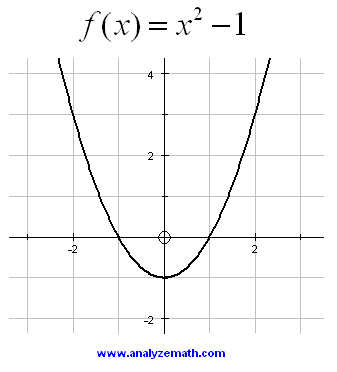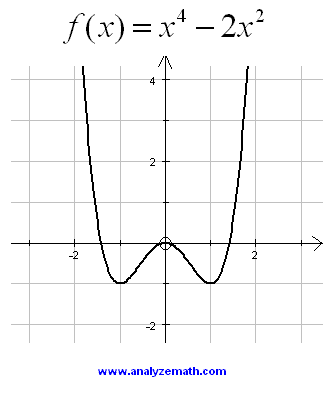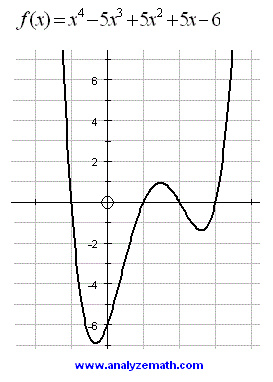# Graphs of Polynomials Functions

 The graphs of several polynomials along with their equations are shown. Polynomial of the first degree.Figure 1: Graph of a first degree polynomial Polynomial of the second degree.Figure 2: Graph of a second degree polynomial Polynomial of the third degree.Figure 3: Graph of a third degree polynomial Polynomial of the fourth degree.Figure 3: Graph of a fourth degree polynomialFigure 4: Graph of another fourth degree polynomial Polynomial of the fifth degree.Figure 3: Graph of a fifth degree polynomial Polynomial of the sixth degree.Figure 3: Graph of a sixth degree polynomial More references and links to polynomial functions. Polynomial Functions